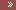THE WORLD OF YOUR POSSIBILITIES - Chapter 2HomePresentationsDATA STRUCTURE NOTES=> Chapter 1=> Chapter 2=> Chapter 3DBMSContactGuestbookLoginToplistCounterTitle of your new pagejhg

Chapter 2: Arrays

2.12      Vectors.

Array definition:-Array is linear, homogeneous data structures whose elements are stored in contiguous memory locations.

Arrays are subscripted variables stored in contiguous memory locations.

Accessing Array elements: Elements of arrays are accessed by using index or subscripts.

Types of Arrays:

One-dimensional Array or linear array: requires only one index to access an element.

Two-Dimensional Array: requires two indices to access an element.

Multidimensional Array: requires two or more indices to access an element.

Size of linear array: size=ub-lb-1

Where ub represents upper bound or largest index of array,

lb represents lower bound or smallest index of array.

Indices of array are integer numbers.

In C/C++/Java index starts from 0,that is the smallest index of array is 0.

In C/C++/Java index are written in brackets [ ].

Representation of One-dimensional array in memory:-

Suppose name of linear array is arr and it has 5 elements. Then its elements are represented as:

arr

0           1           2           3           4

arr, arr, arr, arr, arr.

Since array elements are stored in contiguous memory locations, the computer needs to not to know the address of every element but the address of only first element. The address of first element is called base address of array. Given the address of first element, address of any other element is calculated using the formula:-

Loc (arr [k]) =base (arr) + w * k (in C/C++/Java)

Where k is the index of array whose address we want to calculate and w is the number of bytes per storage location of for one element of array.

If not given explicitly, we take index set as 1,2,3,4,……n where n the upper bound of the array.

Example:- Suppose that array arr is declared as integers with size 20 and its first element is stored at address 1000. Calculate the address of 4th element of array when the index of the array starts from 0.

Thus, loc(arr)=1000 + 2*3=1006

Representation of two dimensional array in memory:

Suppose name of two-dimensional array is mat and it has 3 rows and 4 columns. Then its elements are represented as:

mat,    mat,    mat

mat,     mat[],     mat

mat,    mat,    mat

mat

0                1                      3

 0

 1

 2

Elements of two-dimensional arrays are stored in two ways:-

(i)                     Column major order: Elements are stored column by column, i.e. all elements of first column are stored, and then all elements of second column stored and so on.

 mat

 Column 0
 mat

 mat

 mat

 Column 1
 mat

 mat

 mat

 Column 2
 mat

 mat

(ii)                Row major order: Elements are stored row by row, i.e. all elements of first row are stored, and then all elements of second row stored and so on.

 mat

 Row 0
 mat

 mat

 mat

 Row 1
 mat